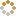﻿ Ningbo University of Technology Online Judge ::  Foxy Information Processing System
• ###  Foxy Information Processing System

• 时间限制: 1000 ms　内存限制: 65535 K
• 问题描述
• Foxy Information Processing System is the Next Generation Information Processing System. We can call it FIPS or NGIPS.
Let's see how foxy it is:
We assume there're two servers with FIPS are receiving information from clients. So there's a message queue. Strictly speaking, there's a message doubly linked list.
Two servers get messages in turns. But they only can get the message in the front or back of message list.
Each FIPS will analyze the amount of information of each message in the list. Then it will get a message in the front or back of message list. For each getting, it will change strategy to make sure that after getting all of the messages in queue it can get the maximum amount of information.

Now give you a message list. Please tell me the amount of information that FIPS I and FIPS II can get.
• 输入
• This problem contains several cases.
The first line of each case is an integer N (1<=N<=1000), indicates the number of messages in list.
Then follow a line with N integers. Each integer indicates the amount of information that each message contains. Each amount is between 4 and 800, and must be the multiple of 4.
We assume that FIPS I takes the first message.
• 输出
• For each case, you should output the amount of information that FIPS I and FIPS II can get.
Leave a blank line between two cases.
• 样例输入
• ```3
4 12 4
4
4 12 16 4
```
• 样例输出
• ```FIPS I : 8
FIPS II: 12

FIPS I : 20
FIPS II: 16
```
• 提示
• `无`
• 来源
• `XadillaX`
• 操作
﻿